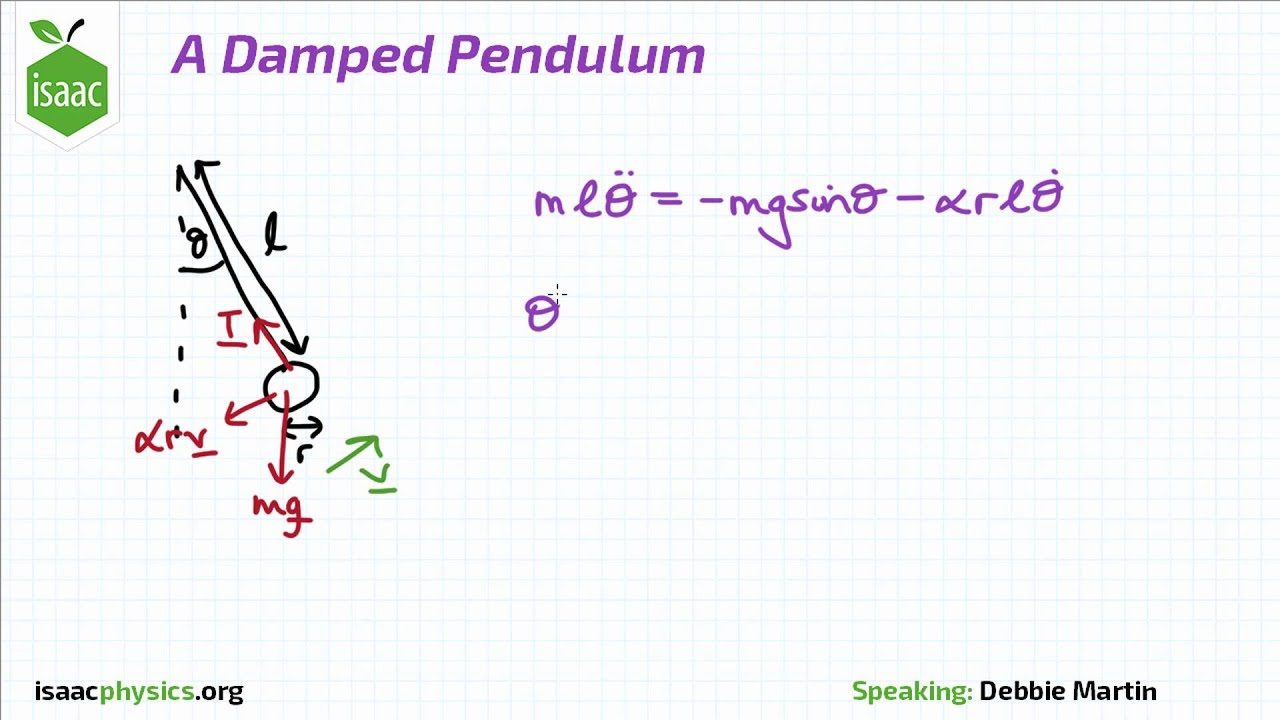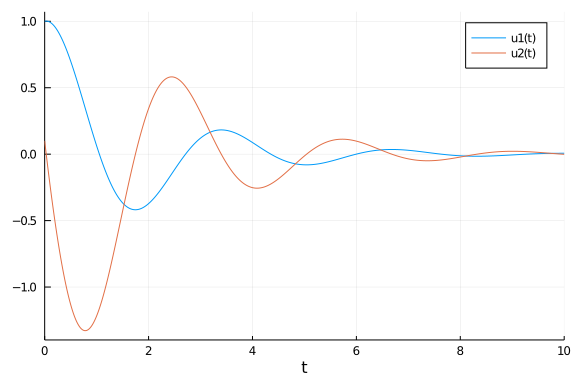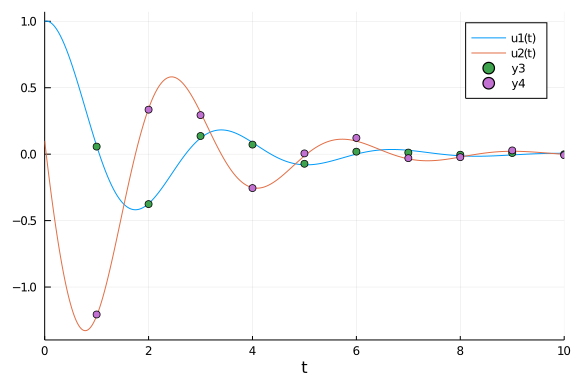# Bayesian Inference on a Pendulum using DiffEqBayes.jl

### Set up simple pendulum problem

using DiffEqBayes, OrdinaryDiffEq, RecursiveArrayTools, Distributions, Plots, StatsPlots, BenchmarkTools, TransformVariables, CmdStan, DynamicHMC


Let's define our simple pendulum problem. Here our pendulum has a drag term ω and a length L.We get first order equations by defining the first term as the velocity and the second term as the position, getting:

function pendulum(du,u,p,t)
ω,L = p
x,y = u
du = y
du = - ω*y -(9.8/L)*sin(x)
end

u0 = [1.0,0.1]
tspan = (0.0,10.0)
prob1 = ODEProblem(pendulum,u0,tspan,[1.0,2.5])

ODEProblem with uType Array{Float64,1} and tType Float64. In-place: true
timespan: (0.0, 10.0)
u0: [1.0, 0.1]


### Solve the model and plot

To understand the model and generate data, let's solve and visualize the solution with the known parameters:

sol = solve(prob1,Tsit5())
plot(sol)It's the pendulum, so you know what it looks like. It's periodic, but since we have not made a small angle assumption it's not exactly sin or cos. Because the true dampening parameter ω is 1, the solution does not decay over time, nor does it increase. The length L determines the period.

### Create some dummy data to use for estimation

We now generate some dummy data to use for estimation

t = collect(range(1,stop=10,length=10))
randomized = VectorOfArray([(sol(t[i]) + .01randn(2)) for i in 1:length(t)])
data = convert(Array,randomized)

2×10 Array{Float64,2}:
0.0569058  -0.375845  0.136638   0.0720362  …  0.00859386  -0.000752689
-1.20656     0.334965  0.293891  -0.255306      0.0281103   -0.00753411


Let's see what our data looks like on top of the real solution

scatter!(data')This data captures the non-dampening effect and the true period, making it perfect to attempting a Bayesian inference.

### Perform Bayesian Estimation

Now let's fit the pendulum to the data. Since we know our model is correct, this should give us back the parameters that we used to generate the data! Define priors on our parameters. In this case, let's assume we don't have much information, but have a prior belief that ω is between 0.1 and 3.0, while the length of the pendulum L is probably around 3.0:

priors = [Uniform(0.1,3.0), Normal(3.0,1.0)]

2-element Array{Distributions.Distribution{Distributions.Univariate,Distrib
utions.Continuous},1}:
Distributions.Uniform{Float64}(a=0.1, b=3.0)
Distributions.Normal{Float64}(μ=3.0, σ=1.0)


Finally let's run the estimation routine from DiffEqBayes.jl with the Turing.jl backend to check if we indeed recover the parameters!

bayesian_result = turing_inference(prob1,Tsit5(),t,data,priors;num_samples=10_000,
syms = [:omega,:L])

Chains MCMC chain (9000×15×1 Array{Float64,3}):

Iterations        = 1:9000
Thinning interval = 1
Chains            = 1
Samples per chain = 9000
parameters        = L, omega, σ
internals         = acceptance_rate, hamiltonian_energy, hamiltonian_energy
_error, is_accept, log_density, lp, max_hamiltonian_energy_error, n_steps,
nom_step_size, numerical_error, step_size, tree_depth

Summary Statistics
parameters      mean       std   naive_se      mcse         ess      rhat

Symbol   Float64   Float64    Float64   Float64     Float64   Float64

L    2.4780    0.2113     0.0022    0.0032   3799.5678    1.0001

omega    1.0897    0.2204     0.0023    0.0042   2820.5866    0.9999

σ    0.1596    0.0382     0.0004    0.0006   4229.9547    1.0000

Quantiles
parameters      2.5%     25.0%     50.0%     75.0%     97.5%
Symbol   Float64   Float64   Float64   Float64   Float64

L    2.0473    2.3485    2.4795    2.6056    2.9082
omega    0.7743    0.9501    1.0518    1.1849    1.6446
σ    0.1006    0.1332    0.1537    0.1796    0.2518


Notice that while our guesses had the wrong means, the learned parameters converged to the correct means, meaning that it learned good posterior distributions for the parameters. To look at these posterior distributions on the parameters, we can examine the chains:

plot(bayesian_result)